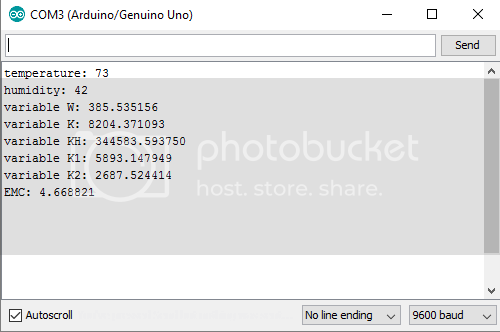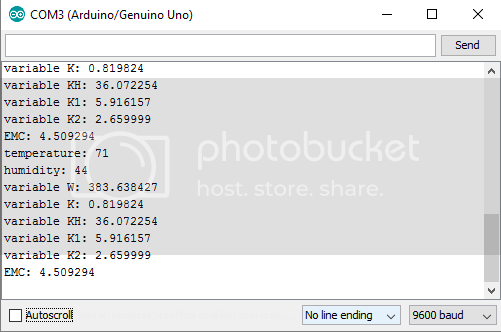# Calculation using sensor data. What am I doing wrong?

Hello all. This is my first post on this forum. I'll get right to it.

I'm working on a hot box for speeding the drying time of wood. I need to calculate the equilibrium moisture content the wood will reach using temperature and humidity data from my sensor(sh7021). I'm using the formula from here, and my code is attached below. I've made some changes to the code to work around the fact that there's only six points of precision available after the decimal.

My problem is that as far as I can tell, I have the formula implemented properly but get a consistent result of around 4.7% during testing regardless of sensor input. In my test environment, the moisture content reading should be 8.2% to 8.5% but I've tested up to 17% and still get the same result of 4.7% I'm not sure if its something I did wrong, or a limitation of the hardware( arduino uno ), but I'd really like to get it sorted out.

``````#include <Wire.h>
#include <SI7021.h>

SI7021 sensor;

void setup() {

sensor.begin();
Serial.begin(9600);
}

void loop() {

si7021_env data = sensor.getHumidityAndTemperature();
float T = data.celsiusHundredths/100;
T = (((T / 5) * 9) + 32);
int t = T;
Serial.println(t);

float H = data.humidityBasisPoints/100;
int h = H;
Serial.println(h);

float W = (330.0 + 0.452*T + 0.00415*(T*T));
Serial.println(W,6);
float K = (7910 + 4.63*T - 0.00844*(T*T));
Serial.println(K,6);
float KH = K*H;
Serial.println(KH,6);
float K1 = (6340 + 0.775*T - 0.0935*(T*T));
Serial.println(K1,6);
float K2 = (1090 + 028.4*T - 0.0904*(T*T));
Serial.println(K2,6);
//float EMC = (1800.0/W * ( KH/(1-KH) + ((K1*KH + 2*K1*K2*K*K*H*H) / (1 + K1*KH + K1*K2*K*K*H*H)) ));
float m1 = KH/(1-KH);
float m2 = (K1*KH + 2*K1*K2*K*K*H*H);
float m3 = (1 + K1*KH + K1*K2*K*K*H*H);
float EMC2 = 1800/W * (m1 + (m2 / m3));
//Serial.println(EMC,6);
Serial.println(EMC2,6);
delay(60000);

}
``````

Could you please append some of your serial output, so we can see what's going on there? You should also add labels to the prints, like this:

``````Serial.print("temperature: ");
Serial.println(t);
``````

...so you know what belongs to what.

Here's a screenshot of the serial output. Code is the same except for the labels you asked for.I reverted the code to use the unedited emc formula. The reason I changed it in the first place is because I assumed the error was due to float only being precise to 6 or 7 digits after the decimal and some of the constants in the formula have higher precision. That doesn't seem to be the cause since I still get a similar result as I did before.

Here is the serial output after changing it back. And below that is the new code.``````#include <Wire.h>
#include <SI7021.h>

SI7021 sensor;

void setup() {

sensor.begin();
Serial.begin(9600);
}

void loop() {
/* int temperature = sensor.getCelsiusHundredths();
temperature = temperature / 100;
temperature = (((temperature / 5) * 9) + 32);
Serial.println(temperature);
delay(2000);

int humidity = sensor.getHumidityPercent();
Serial.println(humidity);
delay(2000);
*/
si7021_env data = sensor.getHumidityAndTemperature();
float T = data.celsiusHundredths/100;
T = (((T / 5) * 9) + 32);
int t = T;
Serial.print("temperature: ");
Serial.println(t);
float H = data.humidityBasisPoints/100;
int h = H;
Serial.print("humidity: ");
Serial.println(h);
float W = (330.0 + 0.452*T + 0.00415*(T*T));
Serial.print("variable W: ");
Serial.println(W,6);
float K = (.7910 + 0.000463*T - 0.000000844*(T*T));
Serial.print("variable K: ");
Serial.println(K,6);
float KH = K*H;
Serial.print("variable KH: ");
Serial.println(KH,6);
float K1 = (6.34 + 0.000775*T - 0.0000935*(T*T));
Serial.print("variable K1: ");
Serial.println(K1,6);
float K2 = (1.09 + 0.0284*T - 0.0000904*(T*T));
Serial.print("variable K2: ");
Serial.println(K2,6);
//float EMC = (1800.0/W * ( KH/(1-KH) + ((K1*KH + 2*K1*K2*K*K*H*H) / (1 + K1*KH + K1*K2*K*K*H*H)) ));
float m1 = KH/(1-KH);
float m2 = (K1*KH + 2*K1*K2*K*K*H*H);
float m3 = (1 + K1*KH + K1*K2*K*K*H*H);
float EMC2 = 1800/W * (m1 + (m2 / m3));
//Serial.println(EMC,6);
Serial.print("EMC: ");
Serial.println(EMC2,6);
delay(60000);

}
``````

The humidity sensor you are using is accurate to at best +/- 3%, which means that 3 decimal digits of arithmetic precision (0.1%) are more than enough for any calculations you need to do.

The zeros after the decimal point don't count in equations like this, so they are all 3 digit constants.

``````K1 = 6.34 + 0.000775T - 0.0000935T2
K2 = 1.09 + 0.0284T - 0.0000904T2
``````
``````     float T = data.celsiusHundredths/100;
``````

celsiusHundredths (and the humidity variable) is declared as an integer so this will be an integer division which will truncate to degrees.
Force the conversion to be done with floating point:

``````    float T = data.celsiusHundredths/100.;
``````

Pete

I don't know what is wrong. But this debug version might help. It tries different test values of H and T.

``````float T;
float H;

void setup() {
Serial.begin(9600);

for (int t = 10; t < 60; t += 10)
{
for (int h = 20; h < 100; h += 20)
{
T = t;
H = h;

T = (((T / 5) * 9) + 32);
Serial.print("temperature: ");
Serial.println(T);
Serial.print("humidity: ");
Serial.println(H);
float W = (330.0 + 0.452 * T + 0.00415 * (T * T));
Serial.print("variable W: ");
Serial.println(W, 6);
float K = (.7910 + 0.000463 * T - 0.000000844 * (T * T));
Serial.print("variable K: ");
Serial.println(K, 6);
float KH = K * H;
Serial.print("variable KH: ");
Serial.println(KH, 6);
float K1 = (6.34 + 0.000775 * T - 0.0000935 * (T * T));
Serial.print("variable K1: ");
Serial.println(K1, 6);
float K2 = (1.09 + 0.0284 * T - 0.0000904 * (T * T));
Serial.print("variable K2: ");
Serial.println(K2, 6);
//float EMC = (1800.0/W * ( KH/(1-KH) + ((K1*KH + 2*K1*K2*K*K*H*H) / (1 + K1*KH + K1*K2*K*K*H*H)) ));
float m1 = KH / (1 - KH);
float m2 = (K1 * KH + 2 * K1 * K2 * K * K * H * H);
float m3 = (1 + K1 * KH + K1 * K2 * K * K * H * H);
float EMC2 = 1800 / W * (m1 + (m2 / m3));
//Serial.println(EMC,6);
Serial.print("EMC: ");
Serial.println(EMC2, 6);
}
}
}

void loop() {
}
``````

jremington: Ok, so the zeroes between the decimal point and first non-zero digit don't count. Good to
know, thanks.

el_supremo: Thanks for the tip, but adding the decimal after 100 made no discernible difference in the
outcome of the calculation.

aarg: Thanks. I'll try it and let you know.

At this point I'm thinking there's something wrong with the formula itself.

Thanks for all the help guys, but I figured it out. The formula was expecting the humidity as a decimal like .45 for example. My code was entering as a number of percent like 45. Dividing H by 100 solved the issue.